Ex 12.2

Chapter 12 Class 9 Herons Formula
Serial order wise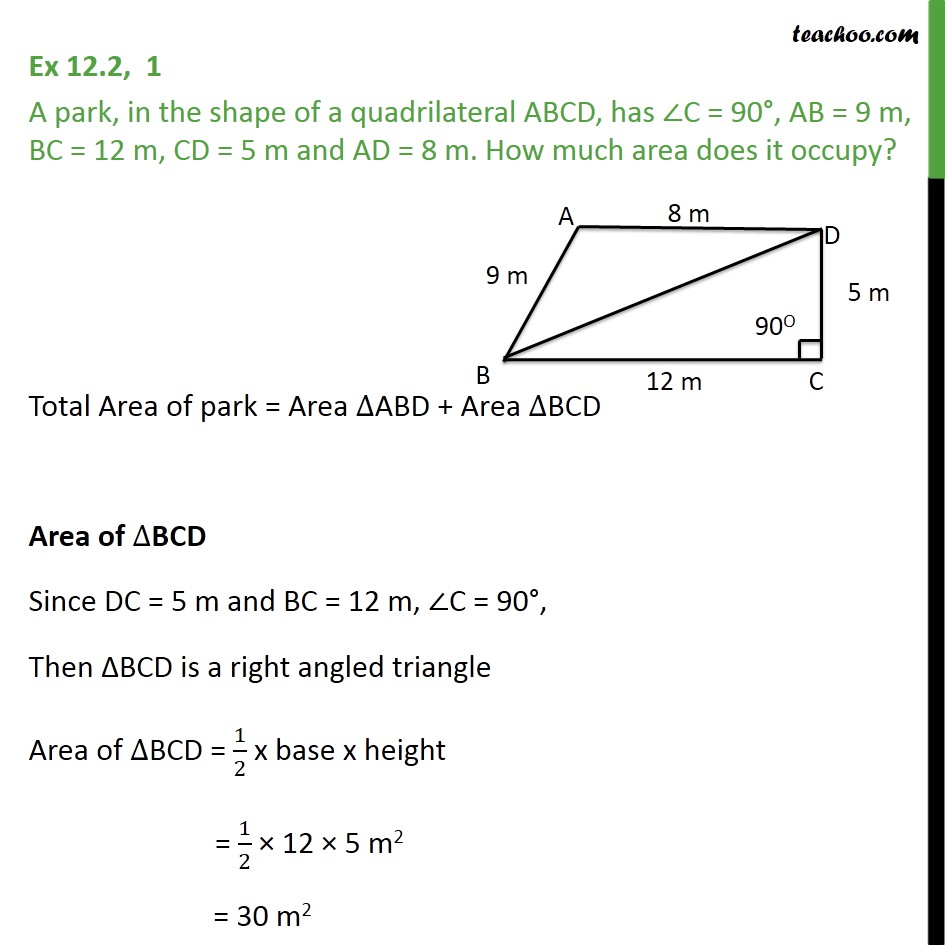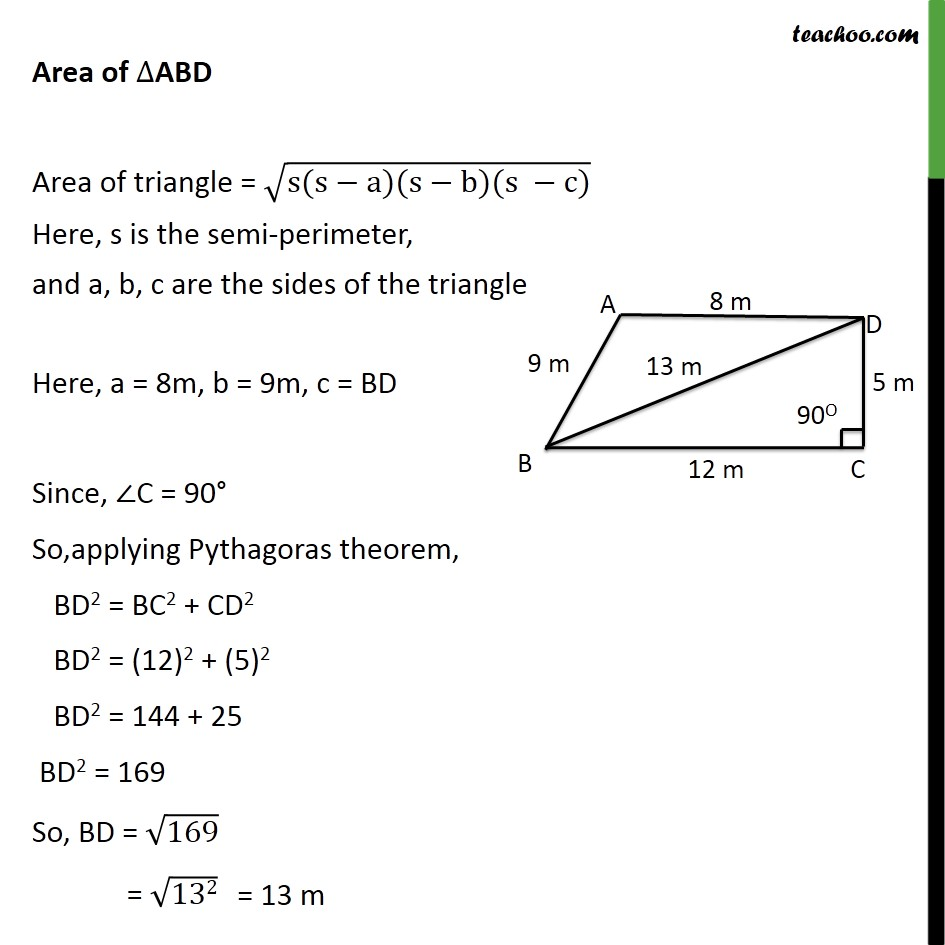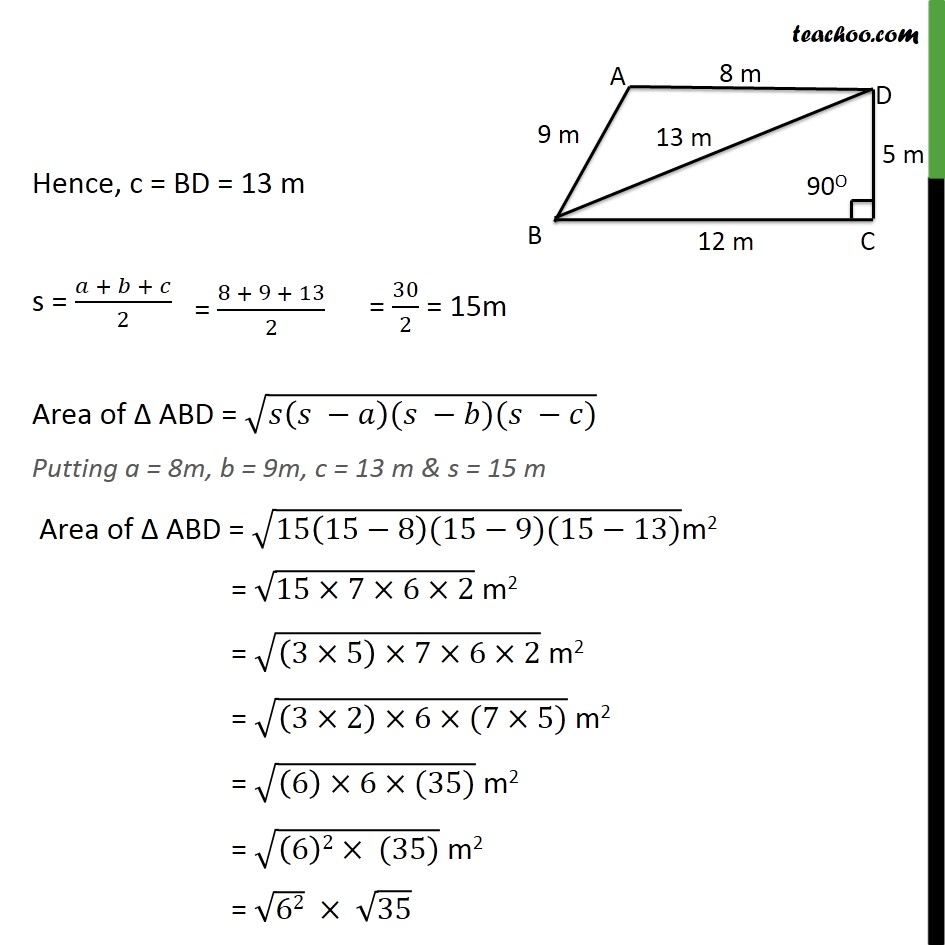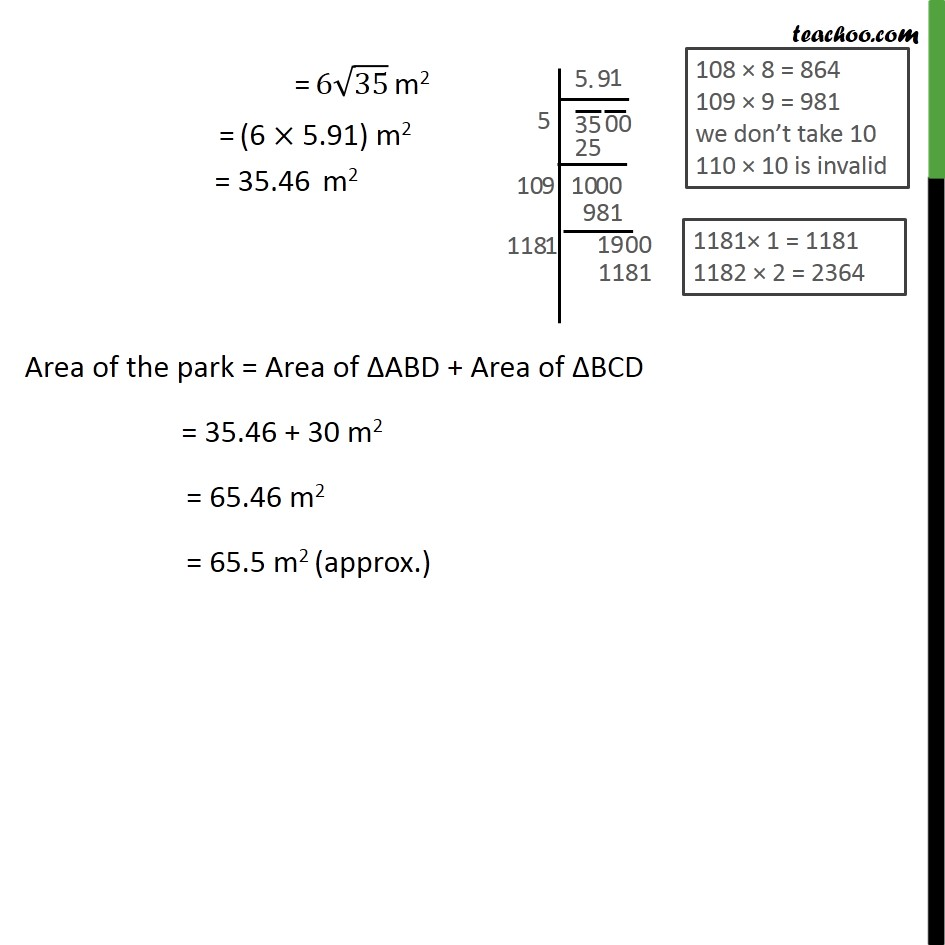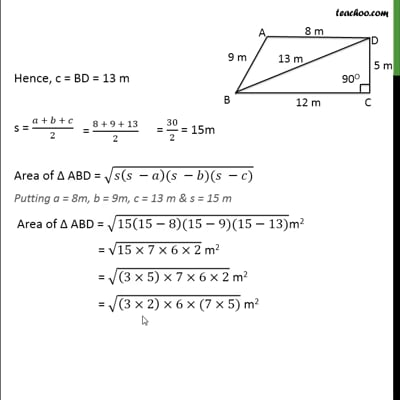This video is only available for Teachoo black users

Solve all your doubts with Teachoo Black (new monthly pack available now!)

### Transcript

Ex 12.2, 1 A park, in the shape of a quadrilateral ABCD, has ∠C = 90°, AB = 9 m, BC = 12 m, CD = 5 m and AD = 8 m. How much area does it occupy? Total Area of park = Area ∆ABD + Area ∆BCD Area of ∆BCD Since DC = 5 m and BC = 12 m, ∠C = 90°, Then ΔBCD is a right angled triangle Area of ∆BCD = 1/2 x base x height = 1/2 × 12 × 5 m2 = 30 m2 Area of ∆ABD Area of triangle = √(s(s−a)(s−b)(s −c)) Here, s is the semi-perimeter, and a, b, c are the sides of the triangle Here, a = 8m, b = 9m, c = BD Since, ∠C = 90° So,applying Pythagoras theorem, BD2 = BC2 + CD2 BD2 = (12)2 + (5)2 BD2 = 144 + 25 BD2 = 169 So, BD = √169 Hence, c = BD = 13 m s = (𝑎 + 𝑏 + 𝑐)/2 Area of Δ ABD = √(𝑠(𝑠 −𝑎)(𝑠 −𝑏)(𝑠 −𝑐)) Putting a = 8m, b = 9m, c = 13 m & s = 15 m Area of Δ ABD = √(15(15−8)(15−9)(15−13))m2 = √(15×7×6×2) m2 = √((3×5)×7×6×2) m2 = √((3×2)×6×(7×5)) m2 = √((6)×6×(35)) m2 = √((6)2× (35)) m2 = √62 × √35 = 6√35 m2 = (6 × 5.91) m2 = 35.46 m2 Area of the park = Area of ΔABD + Area of ΔBCD = 35.46 + 30 m2 = 65.46 m2 = 65.5 m2 (approx.)